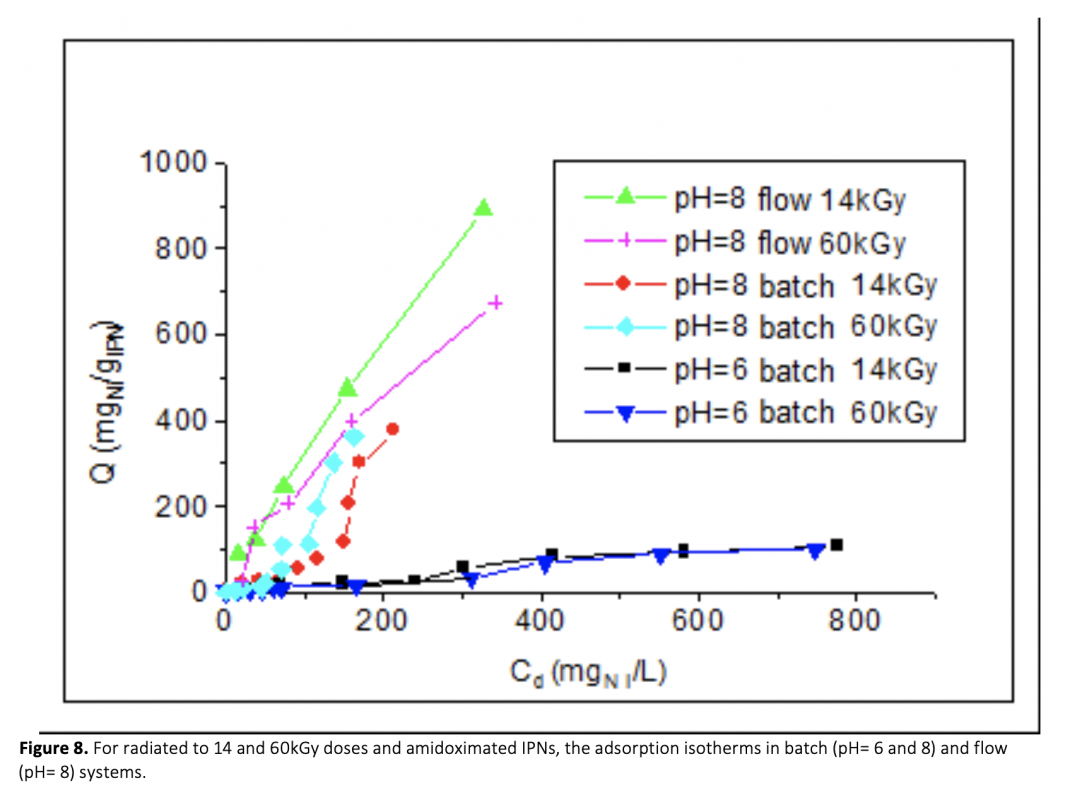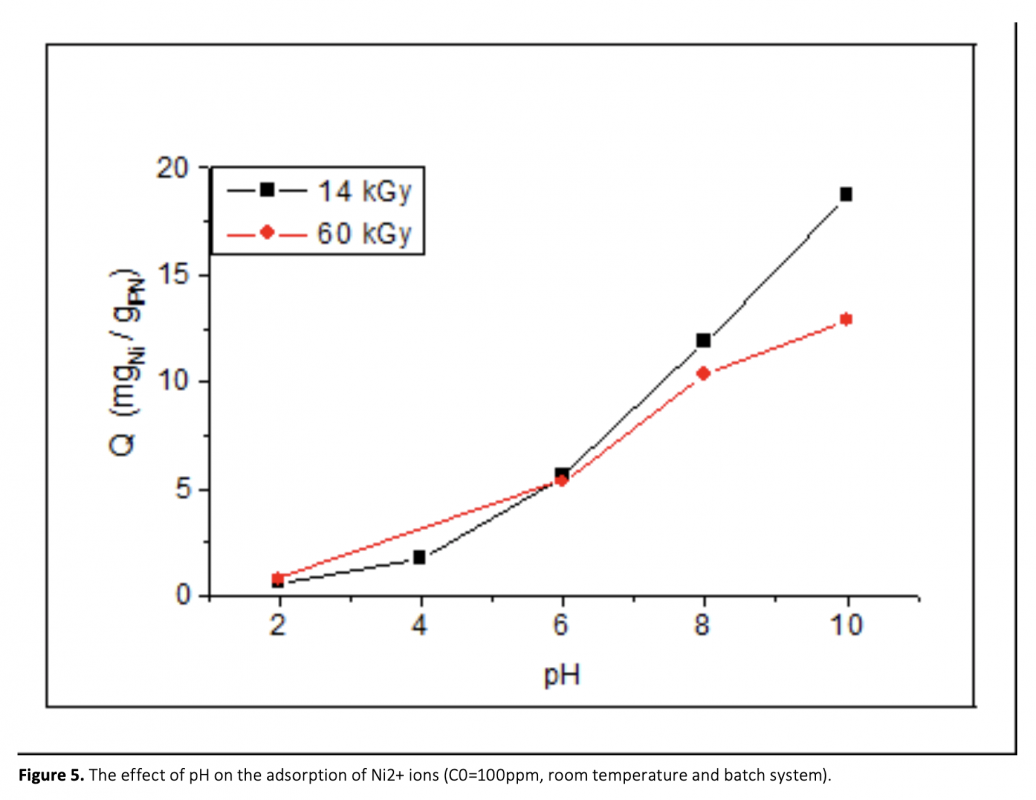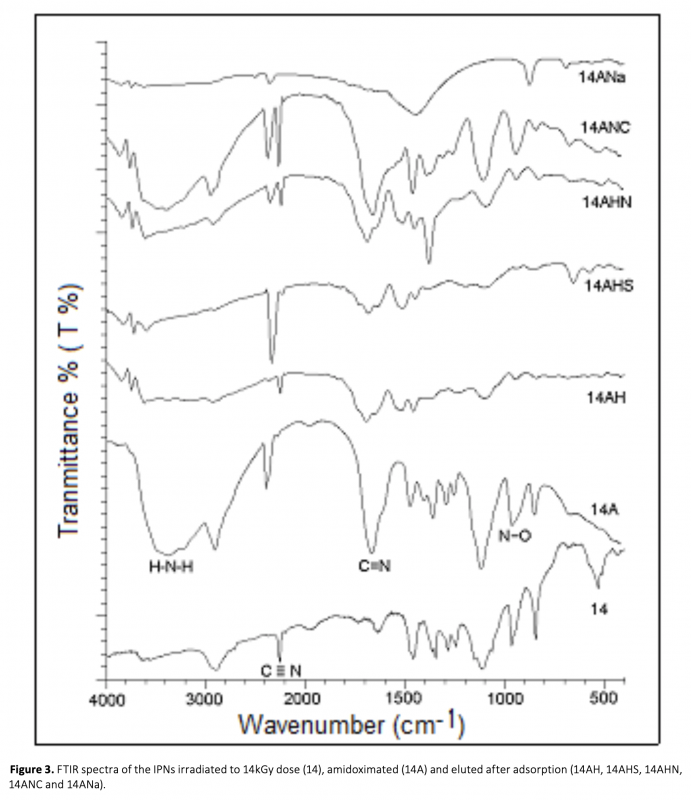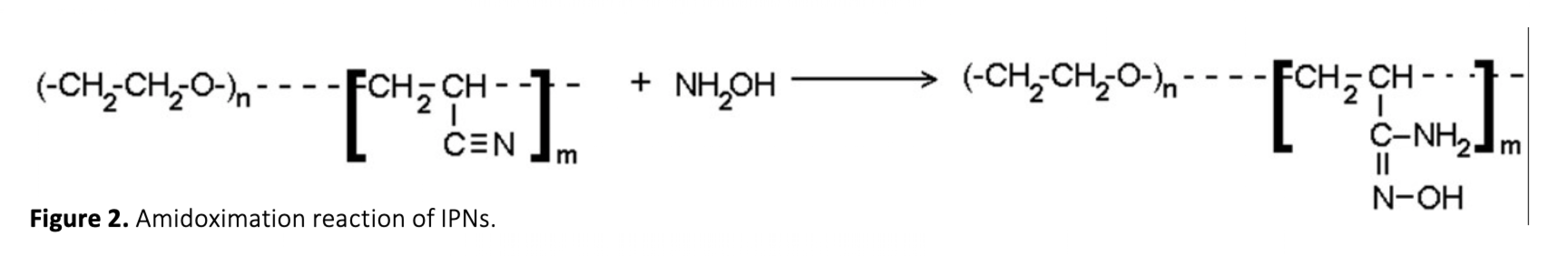#### A Polymeric Adsorbent for Removal And Recovery of Nickel From Aqueous Media

###### Sulu Ortamdan Nikelin Uzaklaştırılması ve Geri Kazanımı Için Polimerik Bir Adsorban

The Interpenetrating Polymer Network (IPN) structure was obtained by gamma radiation of solution of polyethylene glycol (PEG) (35000MW) in an acrylonitrile (AN) to 14 and 60kGy doses were amidoximated with hydroxylamine solution at optimum amidoximation conditions. Effects of pH (2-10) and mNi/mIPN ratio (0.002-0.5) in Ni2+ adsorption were examined by batch process and optimum values were determined as 8 and 0.4 for both doses, respectively. Also, the effect of flow rate to Ni2+ adsorption was examined by the reflux flow process with a fixed-bed reactor at room temperature. Optimum flow rate is determined as 6 mL/s at optimum pH. Ni2+ adsorption studies were carried out in batch (pH= 6 and 8) and flow (pH= 8) systems. Maximum adsorption values reached for irradiated to 14 and 60kGy doses and amidoximated IPNs were given as 105 and 99 mgNi/gIPN in batch system (pH=6), 378 and 361 mgNi/gIPN in batch system (pH= 8) and 891 and 673mgNi/gIPN in flow system (pH= 8), respectively. Maximum elution efficiency was obtained for HCl as being 99.5% and 66.1% for IPNs irradiated to 14 and 60kGy doses and amidoximated, respectively. FTIR results showed that HCl caused structural deformation. Minimum elution efficiency was obtained for (NH4)2CO3 as being 60.1% and 58.2% for IPNs irradiated to 14 and 60kGy, respectively, by the minimum deformation.